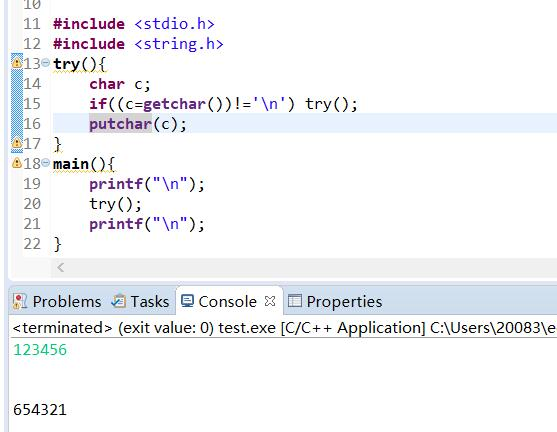C语言的putchar为什么会倒序输出字符串5个回答

try()函数是一个递归，取到回车后，首先putchar打印6，try()函数会返回上一层调用打印5，接着依次打印4321

try()函数里在putchar()之前以调用了try()，这就是递归调用，根据函数栈后进选出的原理，6是最后的，先输出

try()是递归函数``` # include <stdio.h> int main() { int trans(char); char c; printf("输入一串字符串进行翻译:\n"); c = getchar(); while(c != '\n') { c = trans(c); printf("%d", c); getchar(); } putchar('\n'); return 0; } trans(char ch) { if(ch >= 65 && ch <= 86 || ch >= 97 && ch <= 118) ch += 4; if(ch >= 87 && ch <= 90 || ch >= 119 && ch <= 122) ch -= 22; return ch; } ``` 这里我运行以后会出现这样的情况： ![图片说明](https://img-ask.csdn.net/upload/201508/07/1438914665_358848.jpg) **就是我只输入了4个字符，结果却输出了5个，这是怎么回事啊？？** 菜鸟正在学习，跪求大神解答！
C语言，字符串匹配问题

C语言整数转字符串输出
#include <stdio.h> #include <string.h> void to_str(n) int n; { char s; int i = 0; if(n<0) { putchar('-'); n = -n; } do { s[i++] = n%10 +'0'; n/=10; } while(n>0); while(i--)putchar(s[i]); } main() { int x,y; scanf("%d",&x); to_str(x); } 这里面的{ s[i++] = n%10 +'0';这行不懂，为什么要加'0'，不加就不行呢，请赐教。

//递归法逆序输出字符串 #include<stdio.h> void reverse(char *sptr); void main() { char s; printf("Enter a line of text:\n"); gets(s); printf("\nThe line printed backward is:\n"); reverse(s); printf("\n"); } void reverse(char *sptr) { if(*sptr=='\0') { return; } else { reverse(++sptr); putchar(*sptr); } } 最后两行中： reverse(++sptr); /这个++sptr使下面的putchar(*sptr)中的sptr地址也加了1是为什么 putchar(*sptr);
C语言 gets函数相关问题
``` #include<stdio.h> #include <stdlib.h> #include<conio.h> #include<String.h> int main() { FILE *fp; char ch; fp=fopen("F:\\啦啦.txt","at+"); /* if((fp=fopen("F:\\啦啦.txt","at+"))==NULL) { printf("Cannot open file strike any key exit!"); getch(); exit(1); } ch=fgetc(fp); while (ch != EOF) { putchar(ch); ch=fgetc(fp); } *///这个过程是进行读数据的测试过程 //接下来进行语法分析 ch = getchar(); //这里回车作为输入结束 FILE *stream = stdin; int stdin_len = strlen(stream->_ptr); stream->_ptr[strlen(stream->_ptr)-2]='\0'; if (stdin_len > 0) { fputs(stream->_ptr-1, fp); //stream->_ptr 后面会带有\n\n 不需要就开空间复制前面的输入字符串 } char en; gets(en); printf("%s",en);//测试gets函数效果 system("pause"); return 0; } ``` 我想测试下gets函数效果，这个函数就是运行的时候 ，等待用户输入，然后我弄了个输出，输出结果很变态![图片说明](https://img-ask.csdn.net/upload/201910/09/1570598808_700634.png) 输出结果 变少了 为什么呢。
C语言项目，功能8，9不能正常实现，求修改，按如图要求

``` #include<stdio.h> #include<string.h> int n; void g(char a[],int n) { int b; b=a[n]; printf("%c",b); if(n!=0) { n--; g(a,n); } } void main() { char a; gets(a); n=strlen(a); g(a,n); putchar('\n'); } ``` 如代码所示，这是一个字符串的回文，但是为什么在主函数中gets会自动加一个空格？例如，我输入abc，a[]={"a","b","c"," ","\0"}。
C语言中字符数组作为函数返回值出现的问题

C语言程序用fputc函数写入字符到建立的文件中，程序运行正常，可是资源管理器里找不到建立的新文件？
//怎样向文件读写字符 #include<stdio.h> #include<stdlib.h> int main() { FILE * fp;//定义指向文件的指针 char ch,filename;//ch代表输入的字符变量，filename代表文件名 printf("请输入所用的文件名:"); scanf("%s",filename);//输入文件名 if((fp = fopen(filename,"w")) == NULL)//打开文件并使fp指向此文件 { printf("无法打开此文件\n");//如果打不开信息出错 exit(0);//终止程序 } ch = getchar();//用来接收最后的回车字符 printf("请输入一个准备存储到磁盘的字符串（以#结束）:"); ch = getchar();//接收从键盘输入的第一个字符 while(ch != '#')//当输入#时结束 { fputc(ch,fp);//向磁盘文件输出一个字符 putchar (ch);//将输出的字符显示在屏幕上 ch = getchar();//再接受从键盘输入的一个字符 } fclose(fp);//关闭文件 putchar (10);//向屏幕输出一个换行符 return 0; }

#include "stdio.h" #include "string.h"//字符串函数 #include "stdlib.h" long Fact(int n); long FactSum(int v); //函数的定义（作用：计算输入阶乘数的阶乘和，并将结果返回主函数 void displaymain()//显示主菜单 { printf("*******************\n"); printf("简易计算器\n"); printf("*******************\n"); printf("请选择\n"); printf("1：加法\n"); printf("2：减法\n"); printf("3：乘法\n"); printf("4：除法\n"); printf("5：求余\n"); printf("6：累加\n"); printf("7：阶乘\n"); printf("8：阶乘累加\n"); printf("9：退出系统\n"); } void password() { int i=0,temp; char password={0}; //声明一个最多有十个元素的数组，并且初始化值为0； printf("请输入密码："); scanf("%s",password);//输入密码（字符串） if(strcmp("19491001",password)==0)//strcmp:比较两个字符串是否一样 { printf("登陆成功\n"); int choice,flag=0; void displaymain(); void plus(); void minus(); void multiply(); void divide(); void complementation(); void accumulation(); int factorial(); int factorialSum(); void tuichu(); while(flag==0) { putchar('\n'); displaymain(); printf("please input a digital(1-7)\n"); scanf("%d",&choice); if(choice>=1&&choice<=7) switch(choice) { case 1:plus();break; case 2:minus();break; case 3:multiply();break; case 4:divide();break; case 5:complementation();break; case 6:accumulation();break; case 7:factorial();break; case 8:factorialSum();break; case 9:tuichu();break; default:printf("选项输入错误!\n"); } } getch(); //菜单 } else { printf("密码错误\n"); } } void plus() { double a,b,temp; printf("请输入以空格分隔的两个运算数：\n"); scanf("%lf %lf",&a,&b); temp=a+b; printf("结果是：%lf",temp); } void minus() { double a,b,temp; printf("请输入被减数与减数（并用空格隔开）：\n"); scanf("%lf %lf",&a,&b); temp=a-b; printf("结果是：%f",temp); } void multiply() { double a,b,temp; printf("请输入以空格分隔的两个运算数：\n"); scanf("%lf %lf",&a,&b); temp=a*b; printf("结果是：%f",temp); } void divide() { double a,b,temp; printf("请输入被除数与除数（并用空格隔开）：\n"); scanf("%lf %lf",&a,&b); if(b!=0) { temp=a/b; printf("结果是：%f",temp); } else printf("错误！\n"); } void complementation() { int a,b,temp; printf("请输入两个用空格隔开的运算数：\n"); scanf("%d %d",&a,&b); if(b!=0) { temp=a%b; printf("结果是：%d",temp); } else printf("错误！\n"); } void accumulation() { int n,temp; int a,sum=1; printf("请输入要求累加的数值：\n"); scanf("%d",&n); for(a=2;a<=n;a++) { sum+=a; } temp=sum; printf("累加和为：%d\n",temp); } int factorial() { int m; long ret,temp; printf("请输入想求的阶乘数:"); scanf("%d",&m); ret = Fact(m);//调用函数Fact(),并将函数的返回值存入ret if(ret==-1) printf("输入错误!\n"); else { temp=ret; printf("结果是：%d",temp); } return 0; } long Fact(int n)//函数的定义（作用：计算输入阶乘数的阶乘，并将结果返回主函数,且当n<0时，返回-1） { int i; long result = 1; if(n<0) { return -1; } else { for(i = 2;i<=n;i++) { result*=i; } return result; } } int factorialSum() { int m; long ret,temp; printf("请输入想求的阶乘和数:"); scanf("%d",&m); ret = FactSum(m);//调用函数Fact(),并将函数的返回值存入ret if(ret==-1) printf("输入错误!\n"); else temp=ret; return 0; } long FactSum(int v)//函数的定义（作用：计算输入阶乘数的阶乘和，并将结果返回主函数,且当n<0时，返回-1） { int i; long W=1,j = 1; if(v<0) { return -1; } else { for(i = 2;i<=v;i++) { j*=i; W+=j; } return W; } } void tuichu() { printf("已退出"); } void main() { int temp; password(); printf("结果是：%lf",temp); } ![图片说明](https://img-ask.csdn.net/upload/201911/25/1574687755_948559.png)

#include <stdio.h> #include <string.h> int main () { char ch; int m=0,n=0,p=0,count = 0; FILE *fp = fopen("STRING.txt", "w+"); if (fp!=NULL) { printf("请输入要保存的字符串：\n"); ch = getchar(); while (ch!='\n') { count++; fputc(ch, fp); ch = getchar(); } printf("本次一共写入了%d个字符\n",count); printf("\n"); rewind(fp); ch = fgetc(fp); while (ch!=EOF) { putchar(ch); ch = fgetc(fp); if (ch>='0'&&ch<='9') m++; else if (ch>='A'&&ch<='Z'||ch>='a'&&ch<='z') n++; else p++; } printf("\n"); printf("数字有%d个\n字母有%d个\n其他字符有%d个\n",m,n,p); } fclose(fp); return 0; }
C语言课后题，答案不对？

C语言求解，简单问题求解

//怎样向文件读写字符，本体我的问题是为什么没有用fgetc函数逐渐输入字符，还有为什么我在资源管理器里找不到新建的文件？ #include<stdio.h> #include<stdlib.h> int main() { FILE * fp; char ch,filename; printf("请输入所用的文件名:"); scanf("%s",filename); if((fp = fopen("filename","w")) == NULL) { printf("无法打开此文件\n"); exit(0);//终止程序 } ch = getchar();//这个语句不理解， printf("请输入一个准备存储到磁盘的字符串（以#结束）:"); ch = getchar(); while(ch != '#') { fputc(ch,fp); putchar (ch); ch = getchar(); } fclose(fp); putchar (10); return 0; }

//怎样向文件读写字符 #include<stdio.h> #include<stdlib.h> int main() { FILE * fp; char ch,filename； printf("请输入所用的文件名:"); scanf("%s",filename); if((fp = fopen(filename,"w")) == NULL)//为什么这里的filename没有双引号引起来； { printf("无法打开此文件\n"); exit(0);//终止程序 } ch = getchar();//这个语句不理解 printf("请输入一个准备存储到磁盘的字符串（以#结束）:"); ch = getchar(); while(ch != '#') { fputc(ch,fp); putchar (ch); ch = getchar(); } fclose(fp); putchar (10); return 0; }
Segmentation fault问题
//c语言实现输入任意长度的字符串 #include <stdio.h> #include <stdlib.h> int main (void ) { char *string = NULL ,*temp = NULL; char ch; int i; //先将字符串初始化为空 string = (char *)malloc(1); string = '\0'; //len为实际长度(包括空字符) int len = 1; while ((ch = getchar())!= '\n') { //申请一个新字符串 temp = (char *)malloc(len+1); if (temp = NULL) { printf("内存不足！"); free(string); exit(0); } i=0; while (string[i] != '\0'&&i<len) { //把原字符串复制到新字符串 temp[i] = string[i]; i++; } //把新字符归位 temp[i] = ch; //把空字符归位 temp[i+1] = '\0'; //释放源字符，这一步很关键，否 //则会造成内存泄露 free(string); string = temp; len++; } int j=0; while (string[j]!='\0') { putchar(string[j]); j++; } return 0; } 运行时显示Segmentation fault怎么办？

C语言对用堆导入并显示的数据进行进一步的处理

《奇巧淫技》系列-python！！每天早上八点自动发送天气预报邮件到QQ邮箱

YOLO 是我非常喜欢的目标检测算法，堪称工业级的目标检测，能够达到实时的要求，它帮我解决了许多实际问题。 这就是 YOLO 的目标检测效果。它定位了图像中物体的位置，当然，也能预测物体的类别。 之前我有写博文介绍过它，但是每次重新读它的论文，我都有新的收获，为此我准备写一个系列的文章来详尽分析它。这是第一篇，从它的起始 YOLOv1 讲起。 YOLOv1 的论文地址：https://www.c

20行Python代码爬取王者荣耀全英雄皮肤

2019年互联网寒冬，大批企业开始裁员，下图是网上流传的一张截图： 裁员不可避免，那如何才能做到不管大环境如何变化，自身不受影响呢？ 我们先来看一个有意思的故事，如果西游记取经团队需要裁员一名，会裁掉谁呢，为什么？ 西游记团队组成： 1.唐僧 作为团队teamleader，有很坚韧的品性和极高的原则性，不达目的不罢休，遇到任何问题，都没有退缩过，又很得上司支持和赏识(直接得到唐太宗的任命，既给
Python语言高频重点汇总
Python语言高频重点汇总 GitHub面试宝典仓库——点这里跳转 文章目录Python语言高频重点汇总**GitHub面试宝典仓库——点这里跳转**1. 函数-传参2. 元类3. @staticmethod和@classmethod两个装饰器4. 类属性和实例属性5. Python的自省6. 列表、集合、字典推导式7. Python中单下划线和双下划线8. 格式化字符串中的%和format9.

ES6基础-ES6的扩展

Python爬虫爬取淘宝，京东商品信息

Java工作4年来应聘要16K最后没要,细节如下。。。

Python爬虫精简步骤1 获取数据

CPU对每个程序员来说，是个既熟悉又陌生的东西？ 如果你只知道CPU是中央处理器的话，那可能对你并没有什么用，那么作为程序员的我们，必须要搞懂的就是CPU这家伙是如何运行的，尤其要搞懂它里面的寄存器是怎么一回事，因为这将让你从底层明白程序的运行机制。 随我一起，来好好认识下CPU这货吧 把CPU掰开来看 对于CPU来说，我们首先就要搞明白它是怎么回事，也就是它的内部构造，当然，CPU那么牛的一个东

2020年1月17日，国家统计局发布了2019年国民经济报告，报告中指出我国人口突破14亿。 猪哥的朋友圈被14亿人口刷屏，但是很多人并没有看到我国复杂的人口问题：老龄化、男女比例失衡、生育率下降、人口红利下降等。 今天我们就来分析一下我们国家的人口数据吧！ 更多有趣分析教程，扫描下方二维码关注vx公号「裸睡的猪」 即可查看！ 一、背景 1.人口突破14亿 2020年1月17日，国家统计局发布
web前端javascript+jquery知识点总结
Javascript javascript 在前端网页中占有非常重要的地位，可以用于验证表单，制作特效等功能，它是一种描述语言，也是一种基于对象（Object）和事件驱动并具有安全性的脚本语言 ，语法同java类似，是一种解释性语言，边执行边解释。 JavaScript的组成： ECMAScipt 用于描述: 语法，变量和数据类型，运算符，逻辑控制语句，关键字保留字，对象。 浏览器对象模型（Br
Qt实践录：开篇

B 站上有哪些很好的学习资源?

Web播放器解决了在手机浏览器和PC浏览器上播放音视频数据的问题，让视音频内容可以不依赖用户安装App，就能进行播放以及在社交平台进行传播。在视频业务大数据平台中，播放数据的统计分析非常重要，所以Web播放器在使用过程中，需要对其内部的数据进行收集并上报至服务端，此时，就需要对发生在其内部的一些播放行为进行事件监听。 那么Web播放器事件监听是怎么实现的呢？ 01 监听事件明细表 名
3万字总结，Mysql优化之精髓

1. 传统事件绑定和符合W3C标准的事件绑定有什么区别？ 传统事件绑定 &lt;div onclick=""&gt;123&lt;/div&gt; div1.onclick = function(){}; &lt;button onmouseover=""&gt;&lt;/button&gt; 注意： 如果给同一个元素绑定了两次或多次相同类型的事件，那么后面的绑定会覆盖前面的绑定 （不支持DOM事...